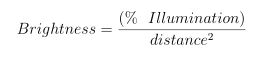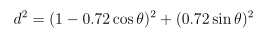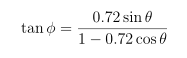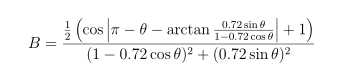# Case study: When is Venus brightest?

Venus changes brightness very rapidly as it approaches inferior conjunction (passes between the earth and the sun.) It is invisible at conjunction, but is at is brightest just 36 days later. Then it dims gradually over the course of almost a year. Why?

There are two major factors that affect how bright Venus appears in the evening (or, starting in March 2017, morning) sky. One is Venus’s distance from the earth. Just like any other light source, the intensity of the light drops off with the square of distance. The other is the phase of Venus. Like the moon, Venus has phases. Venus is “full” when it is on the opposite of the sun from the Earth and “new” when it passes between the Earth and the sun. Just as the full moon is much brighter than the crescent moon, a full Venus would be brighter than a crescent Venus, if viewed from the same distance.

These two factors fight against each other. Venus is full when farthest away, and new when closest. Which factor wins?

## Building a mathematical model

We begin with a simplifying assumption, that Venus is always the same distance from the sun. (The distance actually varies by about 1%.) Venus is, on average, about 108 million km from the sun, or 0.72 AU. It’s very convenient for us to work with astronomical units: for our mathematical model, we will place the sun at the origin, the Earth at (1,0), and move Venus around a circle with radius 0.72. We will need names for two angles: how far Venus is around its circular orbit, theta (θ), and how far Venus appears to be from the sun, as viewed from the Earth, phi (φ).

Our basic model is:Calculating distance is the easy part: given θ, we use the Pythagorean theorem to findFinding what fraction of Venus’s surface is illuminated, as viewed from Earth, is a little bit harder. In our sketch above, when θ=φ, Venus is completely dark; when θ=180° and φ=0°, Venus is fully illuminated; in between, the difference between π-θ and ψ describes how much of Venus’s surface will appear to be illuminated from Earth.

We need an equation to tell us what φ corresponds to each θ:and the fraction of Venus’s disc that appears illuminated from earth is proportional to the cosine of the difference between the angles. Putting it all together:## Using the model to get answers

To find out when how Venus’s brightness varies with time, we just have to plot this function as θ goes from 0 to 2π. To find when Venus is brightest, we find the value of θ that maximizes B. This is very easily done in just a few lines of Mathematica code:

``` dist[th_] := Sqrt[((1 - 0.72 Cos[th])^2 + (0.72 Sin[th])^2)]; illum[th_] := (Cos[Abs[(Pi - th) - phi[th]]] + 1)/2; phi[th_] := ArcTan[(0.72 Sin[th])/(1 - 0.72 Cos[th])]; bright[th_] := illum[th] / dist[th]^2; ```

We can plot how each of these varies through Venus’s orbital cycle. A complete orbital cycle from θ=0 to θ=2π takes 584 days. (It takes Venus 224.65 days to circle the sun, and takes Earth 365.25 days. In 584 days, Venus “laps” us, making 2.59 circuits to our 1.59.)

We can find exactly when the maxima and minima occur:
``` FindMaximum[phi[th], {th, 0.9}] ```

```{0.803802, {th -> 0.766994}} ```
The maximum of φ is called greatest eastern (or western) elongation: our model says that Venus is never more than 0.804 radians = 46 degrees away from the sun in the sky, and that greatest elongation happens .767(584)/(2π) = 71 days after inferior conjunction. (In the real world, it varies from 45° up to 47.8°, since Venus varies from 0.718 to 0.728 AU from the sun, and earth varies from .983 to 1.017 AU.)

Similarly, we can find the time of maximum brightness:
``` FindMaximum[bright[th], {th, 0.5}] ```

`{1.40903, {th -> 0.396053}}`

``` bright[Pi] 0.338021 %%[] / % 4.16849 Log[2.512, %20] ```

```1.54987 ```

Our model says that Venus is brightest (.396)(584)/(2π) = 37 days after inferior conjunction. Our model further predicts that Venus at maximum brightness is 4.16 times brighter — 1.54 units on the astronomical magnitude scale — than when it is farthest from the earth. Our time estimate was quite good (most published sources say 36 days) but our magnitude estimate turns out to be quite poor. We assumed that the brightness of Venus was simply proportional to how much of Venus’s disc was illuminated. In fact, Venus is quite a bit dimmer when illuminated only by glancing rays of the sun, and quite a bit brighter when struck directly by sunlight, than we assumed. Examining the Light Curve of Venus as provided by The Sky Live, we see that in fact, Venus is only about 1.0 units of magnitude dimmer when it is on the far side of the sun. It actually becomes slightly brighter again at superior conjunction, because sunlight reflects particularly well off of the cloud tops when the sun shines straight down onto them.

A simple mathematical model of a natural process usually only encapsulates the most essential features of the underlying process; the model can be made more and more realistic by introducing more and more detail to the equations — in our case, a more complicated model for brightness than cos(π-θ-φ).

Here at Excelsior Statistics and Optimization, we are happy to work with you to model a process that interests you in as much detail as you wish for us to. Contact us and tell us about your modeling needs.

Back to maximum likelihood page
Back to main optimization page